# VBA Array SizeArticle byExcelMojo Team## What is VBA Array Size?

In VBA, an array is a data structure that allows you to store multiple values of the same data type under a single variable name. The VBA array size refers to the number of elements stored in an array. It determines the maximum capacity of the array.

Arrays in VBA can have fixed or dynamic sizes. A fixed-size array has a predetermined number of elements specified at the time of declaration, while a dynamic array can change its size during runtime.

###### Key Takeaways
• The size of an array in VBA refers to the number of elements it can hold.
• For fixed-size arrays, the size is determined at compile time and cannot be changed during runtime. Dynamic arrays, on the other hand, can be resized using the ReDim statement.
• The ReDim Preserve statement allows you to resize an array while preserving its existing values.

### How to Find the Size of an Array Using VBA Code?

To find the size of an array using VBA code, you can use the “VBA Array Size UBound and LBound” functions. Here’s a step-by-step guide:

1. Declare and initialize your array.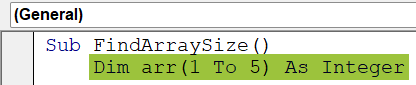2. Determine the size of the array using the UBound and LBound functions.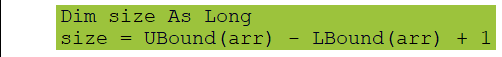The “UBound(arr)” function returns the upper bound (index) of the array, and “LBound(arr)” returns the lower bound (index). By subtracting the lower bound from the upper bound and adding 1, you get the size of the array.

3. Display the size of the array.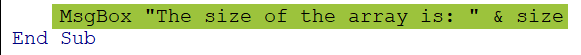The MsgBox function displays a message box with the size of the array.
Running the “FindArraySize” subroutine will display a message box showing the size of the array.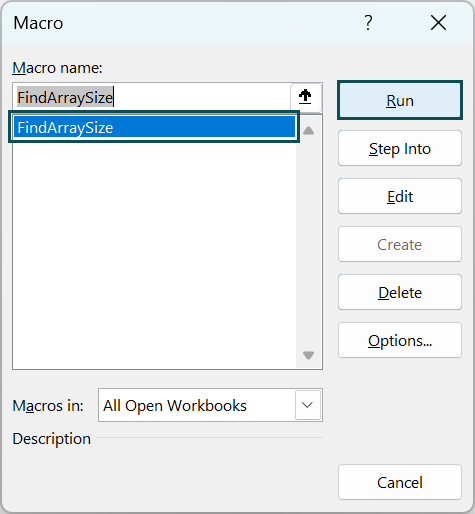In this example, it will display “The size of the array is: 5” since we have declared an array with five elements.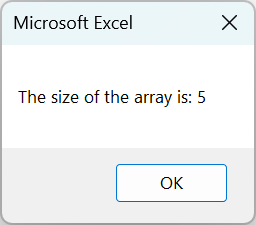Here is the complete code:

Sub FindArraySize()
Dim arr(1 To 5) As Integer ‘ Example array with 5 elements
Dim size As Long
size = UBound(arr) – LBound(arr) + 1
MsgBox “The size of the array is: ” & size
End Sub

### Find Size of an Array Automatically

Finding the size of an array involves determining the number of elements in a dynamically declared array based on the actual values assigned to it. You can use the VBA Array Size Dynamic function for this.

• Step 1: Declare and initialize your dynamic array.
• Step 2: Determine the size of the array using the “UBound” and “LBound” functions.

The “UBound(arr)” function returns the upper bound (index) of the array, and “LBound(arr)” returns the lower bound (index). By subtracting the lower bound from the upper bound and adding 1, you get the size of the array.

• Step 3: Display the size of the array.

The MsgBox function displays a message box with the size of the array.

Running the “FindArraySizeAutomatically” subroutine will display a message box showing the size of the array.

In this example, it will display “The size of the array is: 6” since we have assigned an array with six elements.

Note: The VBA array size refers to the total number of elements in an array while finding the array size refers to dynamically determining an array’s size without explicitly specifying the number of elements.

Here is the complete code:

Sub FindArraySizeAutomatically()
Dim arr() As Variant ‘ Declare a dynamic array
arr = Array(1, 2, 3, 4, 5, 6) ‘ Example array with initial values
Dim size As Long
size = UBound(arr) + 1 ‘ Calculate the size of the array
MsgBox “The size of the array is: ” & size ‘ Display the size of the array
End Sub

### Examples

#### Example #1

This example showcases using VBA Array Size to efficiently store and manipulate data for populating spreadsheet cells.

• Step 1: Open the Visual Basic Editor (VBE).

To open the VBE, press the Alt + F11 keys in Excel or click on the Developer tab in the ribbon and then click on the Visual Basic button.

• Step 2: Create a new module. In the VBE, click the Insert menu and select Module to create a new module.
• Step 3: Start the subroutine “PopulateCellsFromArray.”
• Step 4: Declare an array variable named ‘arr’ with a size of 5 and type String.

This line declares an array variable arr with a size of 5, meaning it can store 5 elements. The elements in this array will be of type String.

• Step 5: Declare a loop counter variable i of type Integer.

This line declares a variable i of type Integer. It will be used as a loop counter in the subsequent loops.

• Step 6: Start a loop to assign values to the array elements.

This line marks the start of a loop that will assign values to the elements of the array arr. The loop will iterate from 1 to 5, inclusive.

• Step 7: Assign a string value to each array element and move to the next iteration of the loop.

This line assigns a string value to each element of the array “arr.” The value assigned to each element combines the string “Value ” and the current value of i.

For example, the first element will be assigned the value “Value 1,” the second element “Value 2”, and so on.

“Next I” indicates the end of the loop. It moves the loop counter “i” to the next value and continues with the next iteration of the loop until it reaches the end condition.

• Step 8: Start a loop to write the array values to Excel cells and move to the next iteration of the loop.

This line marks the start of a loop that will write the values of the array ‘arr’ to Excel cells. The loop will iterate from 1 to 5, inclusive.

• Step 9: Write the value of each array element to a corresponding cell in column A.
• This line writes the value of each array element to a corresponding cell in column A of the active sheet in the Excel workbook. The cell address is determined by concatenating the letter “A” with the current value of “i.”
• For example, during the first iteration, the value of arr(1) will be written to cell A1, during the second iteration arr(2) will be written to cell A2, and so on.
• This code utilizes loops and the Range object to efficiently populate cells with the array values.
• Step 10: Save the module and close the VBA. Now press Alt + F8 to open the Macro menu, select “PopulateCellsFromArray,” and execute the code.
• Step 11: Once you execute the code, you will see that the code initializes an array “arr” with five elements and assigns values to each element using a loop. It then writes the values from the array to Excel cells, where each element is placed in a separate cell in column A.

Here is the complete code:

Sub PopulateCellsFromArray()
Dim arr(1 To 5) As String
Dim i As Integer
‘ Assign values to the array
For i = 1 To 5
arr(i) = “Value ” & i
Next i
‘ Write array values to Excel cells
For i = 1 To 5
Range(“A” & i).Value = arr(i)
Next i
End Sub

#### Example #2

The following VBA code showcases how to use an array to populate Excel cells with the values of the months. It assigns the names of the twelve months to an array and then writes those values to consecutive cells in column A of an Excel worksheet. Please refer to module 2 of the “VBA Array Size” workbook.

• Step 1: In the new module, start the subroutine “PopulateMonthCells.”
• Step 2: Declare an array variable “arr” without specifying its size.

This line declares an array variable “arr” without specifying its size. The Variant type allows the array to hold values of any VBA data type.

• Step 3: Declare a loop counter variable “i” of type Integer.

It will be used as a loop counter in the subsequent loop.

• Step 4: Assign the months to the array.

This line assigns the values of the months to the array, arr. The Array function creates an array and assigns the specified values to it.

• Step 5: Start a loop to write the months to Excel cells.

This line marks the start of a loop that will write the array “arr” values to the Excel cells. The loop will iterate from 0 to the array’s upper bound “arr.” The “UBound” function is used to get the index of the last element in the array.

• Step 6: Write the value of each array element to a corresponding cell in column A and move to the next iteration of the loop.

This line writes the value of each array element to a corresponding cell in column A of the active sheet.

The cell address is determined by concatenating the letter “A” with the current value of “i + 1.” The “+ 1” is added to start writing the values from cell A1 instead of A0.

It then moves the loop counter “i” to the next value and continues with the next iteration of the loop until it reaches the end condition.

• Step 7: Save the module and close the VBA. Now press Alt + F8 to open the Macro menu, select “PopulateMonthCells” and run the code.
• Step 8: Once you click on Run, the code assigns the values of the months to an array using the Array function and then writes those values to Excel cells.  The loop iterates through the array of elements and writes them to consecutive cells in column A of the active sheet.

Here is the complete code:

Sub PopulateMonthCells()
Dim arr() As Variant
Dim i As Integer
‘ Assign the months to the array
arr = Array(“January”, “February”, “March”, “April”, “May”, “June”, “July”, “August”, “September”, “October”, “November”, “December”)
‘ Write the months to Excel cells
For i = 0 To UBound(arr)
Range(“A” & i + 1).Value = arr(i)
Next i
End Sub

### Important Things to Note

1. The VBA Array Size UBound function returns the upper bound or the last index of an array dimension. By adding 1 to the result, you can obtain the size of the array.
2. The UBound function can also be used to find the size of specific dimensions in multi-dimensional arrays by specifying the dimension index.
3. When specifying the VBA array size using a variable, it is crucial to ensure that the variable is of a numeric data type. The VBA array size must be determined using a numeric value assigned to the variable. If the variable does not hold a valid numeric value or is not declared with a numeric data type, it will result in a runtime error.

1. How do I change the size of an array in VBA?

In VBA, you cannot directly change the size of an array once it is declared. You need to either declare a new array with the desired size or use dynamic arrays along with the “ReDim” statement to resize the array.

For example: ReDim arr(1 To newSize)

The “ReDim” statement resizes the array to the specified size.

2. How do I increase the size of an array in VBA?

To increase the size of an array in VBA, you can use the ReDim Preserve statement. This statement allows you to resize the array while preserving the existing values. Here’s the relevant line of code:

ReDim Preserve arr(1 To newSize)

In this line, “arr” is the name of the array, and newSize is the desired new size of the array. The “ReDim Preserve” statement resizes the array to the specified size, preserving the existing values.

3. What is the size limit of an array in VBA?

The available memory resources determine the VBA Array size limit. The maximum size of an array depends on the total amount of memory that can be allocated to your VBA project.

By default, VBA array size can have up to 2^30 – 1 elements (approximately 1 billion elements) in a single dimension. If the array size exceeds the available memory, it may result in an “Out of memory” error.